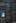20 Oct 2015, Samuel Hinton

# WiggleZ 2D BAO

### Baryon Acoustic Oscillations (BAO) are the sound waves from the primordial soup of energy from the Universe's infancy, frozen out as the Universe expanded. We can see it now in the large scale structure of the universe, and analyse it.

Shall we just jump right into it? The slightly wiggles you can see around $$k=0.1 \rm{h/Mpc}$$ below, when transformed into a correlation function via the Spherical Hankel transformation, turn into that big peak in the right hand plot. As you may have guessed, said “big peak” is the oscillation of interest. Put simply, it represents a characteristic separation at which there is an overdensity of matter in the universe. And its of interest because this peak has existed since the first galaxies formed, and so we can use it as a standard ruler.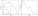Now, it gets even more interesting if we turn the 1D picture above into a 2D picture, but looking not at total separation, but at separation in two specific directions - along our line of sight, and tangential to our line of sight. Our correlation function might just look like this: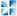I’ve even decomposed the signal into its moments, so that we can see the monopole, quadrupole and hexapole (with their associated spherical Bessel functions), all laid out neatly.

So, my project was essentially to model this 2D BAO signal, and fit it to the WiggleZ data, in order to provide cosmological constraints. The WiggleZ data comes in three different redshift bins, with covariance between data points (shown below) determined courtesy of a set of 600 WizCOLA simulations.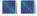We can start a theoretical model using some nice linear software, fix it up slightly (those pesky non-linearities, amongst other things), and then throw our model creation system and the data into a cauldron of MCMC, let it simmer for a million steps or so, and then see what we find.

Here’s one I prepared earlier: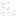I should probably have explained the WiggleZ data is broken down into three different bins. You can combine these bins either before or after fitting, giving the labels “All data” and “Combined” respectively. So, the moral of the story is that we appear to have constrained values on $$\Omega_c h^2$$, $$\alpha$$ and $$\epsilon$$. We can transform these to get us more useful parameters $$\Omega_c h^2$$, $$D_A(z)$$ and $$H(z)$$. As to what values this gives, well, I’ll refer you to my paper, which I will link to, as soon as I write it!